# Calculating Value At Risk In Excel & Python

What is the most I can lose on this investment? This is the question every investor who has invested asks at some point in time. Value at Risk (VaR) tries to provide an answer. In this blog, we understand and compute VaR in Excel and Python using Historical Method and Variance-Covariance approach.### Introduction

VaR was developed in mid-1990s, in response to the various financial crisis, but the origins of the measures lie further back in time.

According to Philippe Jorion, “VaR measures the worst expected loss over a given horizon under normal market conditions at a given level of confidence”.

This definition implies that it is necessary to choose two parameters, namely holding period and confidence level. Typically the confidence level lies in the range 90% to 99% such as 90%, 95%, 99%. Holding period may vary from a day to a year.

Suppose, an analyst says that the 1-day VaR of a portfolio is 1 million dollar, with a 95% confidence level. It implies there is 95% chance that the maximum losses will not exceed 1 million dollar in a single day. In other words, there is only 5% chance that the portfolio losses on a particular day will be greater than 1 million dollar.

There are various methods that are used to calculate the VaR. In this blog, we discuss Variance-Covariance approach and Historical Simulation method.

The Variance-covariance is a parametric method which assumes that the returns are normally distributed. In this method, we first calculate the mean and standard deviation of the returns. For  95% confidence level, VaR is calculated as mean -1.65 * standard deviation and for 99% confidence level, VaR is calculated as -2.33 * standard deviation.

#### Calculating VaR in Excel using Variance-Covariance approach

1. Import the data from Yahoo finance
2. Calculate the returns of the closing price Returns = Today’s Price - Yesterday’s Price / Yesterday’s Price
3. Calculate the mean of the returns using the average function
4. Calculate the standard deviation of the returns using STDEV function
5. Finally, we calculate the VaR for 90, 95, and 99 confidence level using NORM.INV function. This function has three parameters: probability, mean, and standard deviation. In probability, we use 0.1, 0.05, 0.01 respectively for the VaR(90), VaR(95), and VaR(99)

#### Calculating VaR using Python

1. Let us import the necessary libraries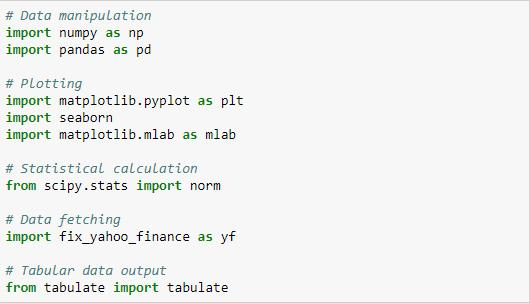2. Import the daily data of stock Facebook from yahoo finance and calculate the daily returns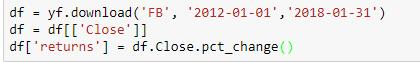3. Determine the mean and standard deviation of the daily returns. Plot the normal curve against the daily returns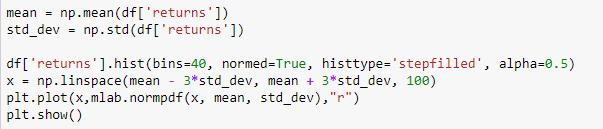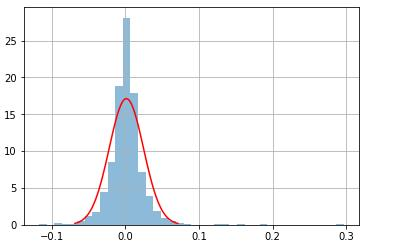4. Calculate the VaR using point percentile function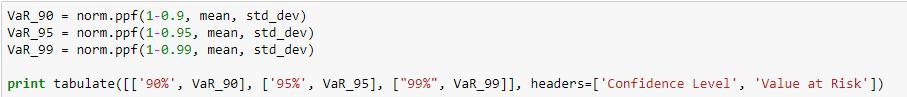Output: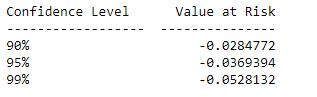#### Historical Simulation approach

The steps followed to calculate the VaR using the historical method in Excel are as follows:

1. Similar to the variance-covariance approach, first we calculate the returns of the stock Returns = Today’s Price - Yesterday’s Price / Yesterday’s Price
2. Sort the returns from worst to best.
3. Next, we calculate the total count of the returns using count function.
4. The VaR(90) is the sorted return corresponding to the 10% of the total count.
5. Similarly, the VaR(95) and VaR(99) is the sorted return corresponding to the 5% and 1% of the total count respectively.

Python:

1. Import the necessary libraries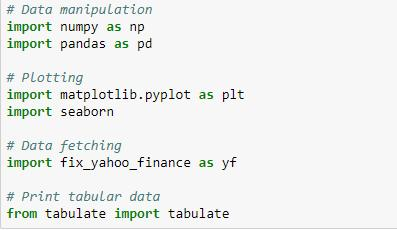2. Calculate the daily returns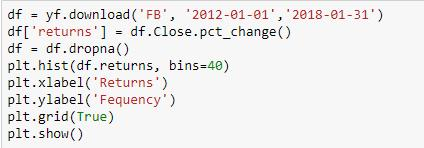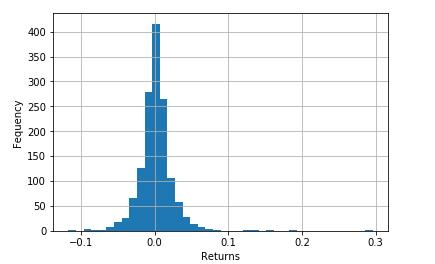3. Sort the returns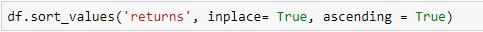4. Calculate the VaR for 90%, 95%, and 99% confidence levels using quantile function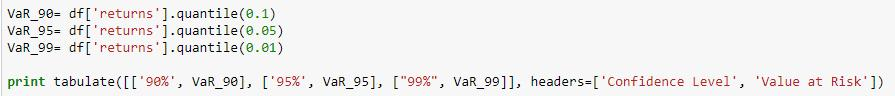Output: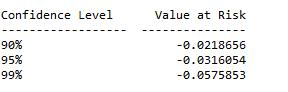As you can see there is a substantial difference in the value-at-risk calculated from historical simulation and variance-covariance approach. This tells us that the return distribution is not normal.

### Conclusion

Here I end this blog but there is one more approach of calculating VaR. If you are interested you can check out the options courses on Quantra which covers different options trading strategies with the risk management techniques.